Test: Chemical Kinetics - 2

# Test: Chemical Kinetics - 2

Test Description

## 30 Questions MCQ Test Physical Chemistry | Test: Chemical Kinetics - 2

Test: Chemical Kinetics - 2 for Chemistry 2022 is part of Physical Chemistry preparation. The Test: Chemical Kinetics - 2 questions and answers have been prepared according to the Chemistry exam syllabus.The Test: Chemical Kinetics - 2 MCQs are made for Chemistry 2022 Exam. Find important definitions, questions, notes, meanings, examples, exercises, MCQs and online tests for Test: Chemical Kinetics - 2 below.
Solutions of Test: Chemical Kinetics - 2 questions in English are available as part of our Physical Chemistry for Chemistry & Test: Chemical Kinetics - 2 solutions in Hindi for Physical Chemistry course. Download more important topics, notes, lectures and mock test series for Chemistry Exam by signing up for free. Attempt Test: Chemical Kinetics - 2 | 30 questions in 90 minutes | Mock test for Chemistry preparation | Free important questions MCQ to study Physical Chemistry for Chemistry Exam | Download free PDF with solutions
 1 Crore+ students have signed up on EduRev. Have you?
Test: Chemical Kinetics - 2 - Question 1

### In a consecutive first order reaction, (where k1 and k2 are the respective rate constants) species B has transient existence. Therefore,

Test: Chemical Kinetics - 2 - Question 2

### The specific rate constant of decomposition of a compound is represented by: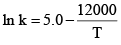The activation energy of decomposition for this compound at 300 K is

Test: Chemical Kinetics - 2 - Question 3

### In a zero-order reaction for every 10° rise of temperature, the rate is doubled. If the temperature is increased from 10°C to 100°C, the rate of the reaction will become

Test: Chemical Kinetics - 2 - Question 4

The fluorescence life time of a molecule in solution is 10 ns. If the fluorescence quantum yield is 0.1, the rate constant of fluorescence decay is

Test: Chemical Kinetics - 2 - Question 5

For the reaction, P + Q + R → S, experimental data for the measured initial rate is given below: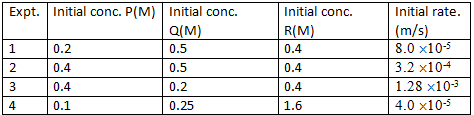The order of the reaction with respect to P, Q and R respectively, is

Test: Chemical Kinetics - 2 - Question 6

Sucrose is converted to a mixture of glucose and fructose in a pseudo first order process under alkaline conditions. The reaction has a half˜life of 28.4 min. The time required for the reduction of a 8.0 mM sample of sucrose to 1.0 mM is:

Test: Chemical Kinetics - 2 - Question 7

The reaction, 2NO(g) + O2(g) →2NO2(g) proceeds via the following steps: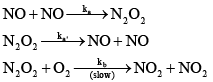The rate of this reaction is equal to

Test: Chemical Kinetics - 2 - Question 8

1 g of 90Sr gas converted to 0.953 g after 2 yr. The half-line of 90Sr, and the amount of 90Sr remaining after 5 yr are:

Test: Chemical Kinetics - 2 - Question 9

How many times will the rate of the elementary reaction 3X + Y → X2Y change if the concentration of the substance X is doubled and that of Y is halved?

Detailed Solution for Test: Chemical Kinetics - 2 - Question 9

Since it is an elementary reaction, its rate law r1 = k [A] 3[B]
When the concentrations are changed the new rate will be r2 = k (2[A])3([B]/2) = 4k[A]3[B]
So, r2 = 4r1.

Test: Chemical Kinetics - 2 - Question 10

A reaction proceeds through the formation of an intermediate B in a unimolecular reaction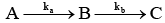:The integrated rate law for this reaction is

Test: Chemical Kinetics - 2 - Question 11

In radical chain polymerization, the quantity given by the rate of monomer depletion, divided by the rate of propagating radical formation is called:

Test: Chemical Kinetics - 2 - Question 12

H2 and Br2 react to give HBr by the following steps: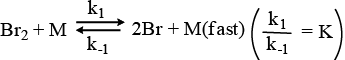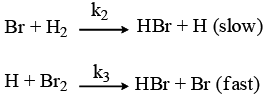The probable rate law for the above sequence is

Test: Chemical Kinetics - 2 - Question 13

The half–line of a order reaction varies with temperature according to:

Detailed Solution for Test: Chemical Kinetics - 2 - Question 13

k = ln2/t1/2 ----(i)

k = Ae(-Ea/RT) ------(ii)

Taking ln on both sides

lnk = ln((ln2)/ t1/2)

lnk = lnA + (-Ea/R) x 1/T

Hence comparing the two equations, we get:

-ln(t1/2) α 1/T

Therefore A is the correct answer.

Test: Chemical Kinetics - 2 - Question 14

The half–life time for a reaction at initial concentrations of 0.1 and 0.4 mol–1 are 200 s and 50 s respectively. The order of the reaction is:

Test: Chemical Kinetics - 2 - Question 15

N2O2(g) and NO(g) react to form NO2 according to the stoichiometric equation

N2O2(g) + NO (g) →3NO2(g) (R1)

At a given temperature and pressure. A possible mechanism for this overall reaction is: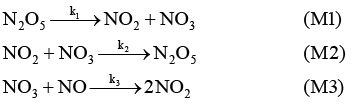Where NO3 is an unstable intermediate. What would be the rate expression of the disappearance of N2O5 in terms of the concentrations of the stable species and the rate constants given above:

Test: Chemical Kinetics - 2 - Question 16

Formation of Z and X is theoretically expected to obey the following kinetic scheme.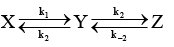An experimentalist wants to verify the above scheme, but can observed and measure the concentration of only X and Z. Is it possible that under certain, the measurements of [X] and [Z] as function of time would lead the experimenter to conclude that the kinetic scheme is as given below, and that the species Y is absent: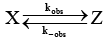Test: Chemical Kinetics - 2 - Question 17

Following is the graph between (a – x)–1 and time t for a second order reaction,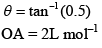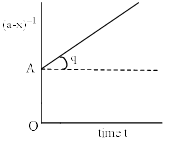Hence, rate at the start of the reaction is

Test: Chemical Kinetics - 2 - Question 18

Which of the following plots represent(s) the Arrhenius rate equation, k=Ae–Ea/RT with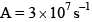and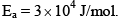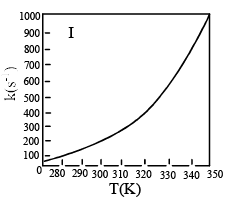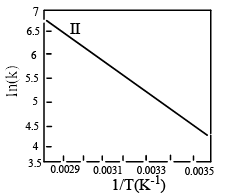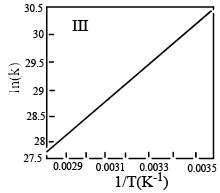Detailed Solution for Test: Chemical Kinetics - 2 - Question 18

Option D is correct as the exponential graph will be there in I and the negative slope will be there in II

Test: Chemical Kinetics - 2 - Question 19

The fraction of group condensed at time t in any stepwise condensation polymerization (overall second order) reaction is

Test: Chemical Kinetics - 2 - Question 20

For the following reaction,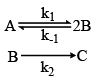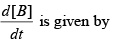Test: Chemical Kinetics - 2 - Question 21

According to Arrhenius equation (K= rate constant and T= Temperature):

Test: Chemical Kinetics - 2 - Question 22

The reaction of nitric oxide with ozone takes place according to the following stoichiometry.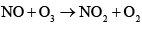The observed rate law for the reaction is found to be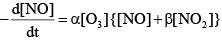Where α and β are constant. To explain the above rate low, the following reaction scheme has been proposed: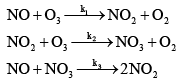Determine an expression for β .

Test: Chemical Kinetics - 2 - Question 23

If the concept of half-life is generalized to quarter–life of a first order chemical reaction, it will e equal to:

Test: Chemical Kinetics - 2 - Question 24

The activation energy for the bimolecular reaction A+BC→AB+C is E0 in the gas phase. If the reaction is carried out in a confined volume of λ3 , the activation energy is expected to:

Detailed Solution for Test: Chemical Kinetics - 2 - Question 24

The minimum energy requirement that must be met for a chemical reaction to occur is called the activation energy. The activation energy depends on nature of the reacting species or reactants. It does not depends on volume that is it will remain constant irrespective of the volume.

So, (A) remain unchanged -- is the correct option

Test: Chemical Kinetics - 2 - Question 25

In a reaction, A+B→Product, rate is doubled when the concentration of B is doubled and the rate increases by a factor of 8 when the concentrations of both the reactants (A and B) are doubled, rate law for the reaction can be written as:

Detailed Solution for Test: Chemical Kinetics - 2 - Question 25

When conc of B is doubled, the rate is doubled. So, Order wrt [B] is 1. The rate increases by a factor of 8 when conc of [A] and [B] are doubled. So Order wrt [A] is 2. Overall Rate is Rate = k[A]2[B]

Test: Chemical Kinetics - 2 - Question 26

The expression for the equilibrium constant (Keq) for the enzyme catalyzed reaction given below is: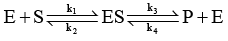Test: Chemical Kinetics - 2 - Question 27

For a reaction involving two steps given below: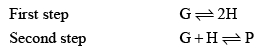Assume that the first step attains equilibrium rapidly. The rate of formation of P is proportional to

Test: Chemical Kinetics - 2 - Question 28

Consider the reaction H2 + C2 H2 → C2 H6 the molecular diameters of H2 and C2H4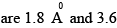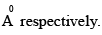The pre-exponential factor in the rate constant calculated using collision theory in m3 (mol) –1 s–1 is approximately (For this reaction at 300K,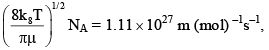where the symbols have their usual meanings):

Test: Chemical Kinetics - 2 - Question 29

A reaction A → B , involves following mechanism: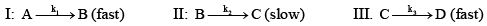The rate law of the reaction may be given as:

Test: Chemical Kinetics - 2 - Question 30

A decomposes as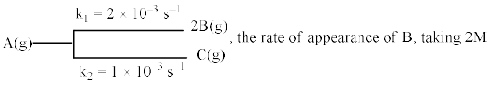Concentration of A, is equal to:

## Physical Chemistry

67 videos|106 docs|31 tests
 Use Code STAYHOME200 and get INR 200 additional OFF Use Coupon Code
Information about Test: Chemical Kinetics - 2 Page
In this test you can find the Exam questions for Test: Chemical Kinetics - 2 solved & explained in the simplest way possible. Besides giving Questions and answers for Test: Chemical Kinetics - 2, EduRev gives you an ample number of Online tests for practice

## Physical Chemistry

67 videos|106 docs|31 tests